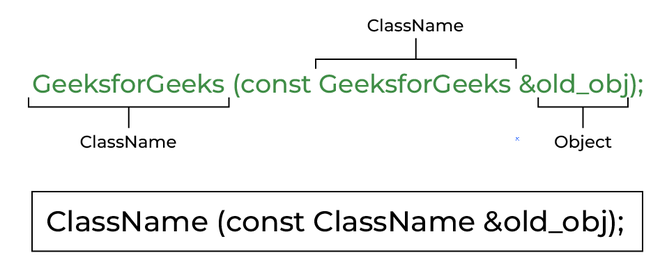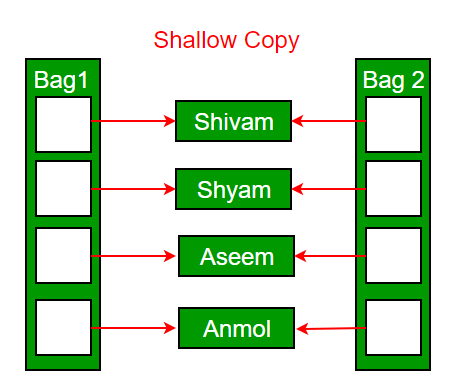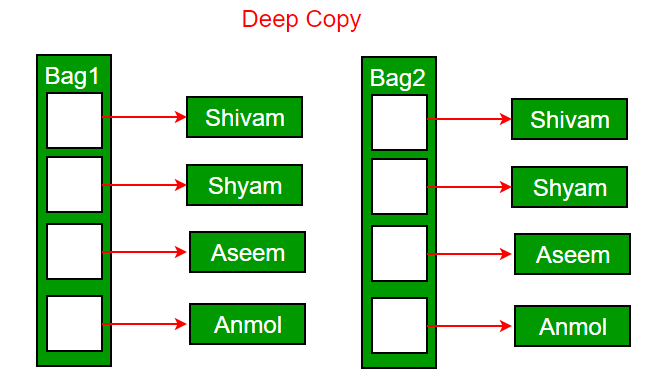Open in App
Not now

## Related Articles

• Write an Interview Experience

# Copy Constructor in C++

• Difficulty Level : Medium
• Last Updated : 05 Jan, 2023

Pre-requisite: Constructor in C++

A copy constructor is a member function that initializes an object using another object of the same class. In simple terms, a constructor which creates an object by initializing it with an object of the same class, which has been created previously is known as a copy constructor.

Example:Syntax of Copy Constructor

## Characteristics of Copy Constructor

1. The copy constructor is used to initialize the members of a newly created object by copying the members of an already existing object.

2. Copy constructor takes a reference to an object of the same class as an argument.

```Sample(Sample &t)
{
id=t.id;
}```

3. The process of initializing members of an object through a copy constructor is known as copy initialization.

4. It is also called member-wise initialization because the copy constructor initializes one object with the existing object, both belonging to the same class on a member-by-member copy basis.

5. The copy constructor can be defined explicitly by the programmer. If the programmer does not define the copy constructor, the compiler does it for us.

Example:

## C++

 `// C++ program to demonstrate the working``// of a COPY CONSTRUCTOR``#include ``using` `namespace` `std;`` ` `class` `Point {``private``:``    ``int` `x, y;`` ` `public``:``    ``Point(``int` `x1, ``int` `y1)``    ``{``        ``x = x1;``        ``y = y1;``    ``}`` ` `    ``// Copy constructor``    ``Point(``const` `Point& p1)``    ``{``        ``x = p1.x;``        ``y = p1.y;``    ``}`` ` `    ``int` `getX() { ``return` `x; }``    ``int` `getY() { ``return` `y; }``};`` ` `int` `main()``{``    ``Point p1(10, 15); ``// Normal constructor is called here``    ``Point p2 = p1; ``// Copy constructor is called here`` ` `    ``// Let us access values assigned by constructors``    ``cout << ``"p1.x = "` `<< p1.getX()``         ``<< ``", p1.y = "` `<< p1.getY();``    ``cout << ``"\np2.x = "` `<< p2.getX()``         ``<< ``", p2.y = "` `<< p2.getY();``    ``return` `0;``}`

Output

```p1.x = 10, p1.y = 15
p2.x = 10, p2.y = 15```

## Types of Copy Constructors

### 1. Default Copy Constructor

An implicitly defined copy constructor will copy the bases and members of an object in the same order that a constructor would initialize the bases and members of the object.

## C++

 `// Implicit copy constructor Calling``#include ``using` `namespace` `std;`` ` `class` `Sample {``    ``int` `id;`` ` `public``:``    ``void` `init(``int` `x) { id = x; }``    ``void` `display() { cout << endl << ``"ID="` `<< id; }``};`` ` `int` `main()``{``    ``Sample obj1;``    ``obj1.init(10);``    ``obj1.display();`` ` `    ``// Implicit Copy Constructor Calling``    ``Sample obj2(obj1); ``// or obj2=obj1;``    ``obj2.display();``    ``return` `0;``}`

Output

```ID=10
ID=10```

### 2. User Defined Copy Constructor

A user-defined copy constructor is generally needed when an object owns pointers or non-shareable references, such as to a file, in which case a destructor and an assignment operator should also be written

## C++

 `// Explicitly copy constructor Calling``#include``using` `namespace` `std;`` ` `class` `Sample``{``    ``int` `id;``    ``public``:``    ``void` `init(``int` `x)``    ``{``        ``id=x;    ``    ``}    ``    ``Sample(){}  ``//default constructor with empty body``     ` `    ``Sample(Sample &t)   ``//copy constructor``    ``{``        ``id=t.id;``    ``}``    ``void` `display()``    ``{``        ``cout<

Output

```ID=10
ID=10```

## C++

 `// C++ Programt to demonstrate the student details``#include ``#include ``using` `namespace` `std;``class` `student {``    ``int` `rno;``    ``string name;``    ``double` `fee;`` ` `public``:``    ``student(``int``, string, ``double``);``    ``student(student& t) ``// copy constructor``    ``{``        ``rno = t.rno;``        ``name = t.name;``        ``fee = t.fee;``    ``}``    ``void` `display();``};``student::student(``int` `no, string n, ``double` `f)``{``    ``rno = no;``    ``name = n;``    ``fee = f;``}``void` `student::display()``{``    ``cout << endl << rno << ``"\t"` `<< name << ``"\t"` `<< fee;``}``int` `main()``{``    ``student s(1001, ``"Ram"``, 10000);``    ``s.display();``    ``student ram(s); ``// copy constructor called``    ``ram.display();``    ``return` `0;``}`

Output

```1001    Ram    10000
1001    Ram    10000```

## When is the copy constructor called?

In C++, a Copy Constructor may be called in the following cases:

• When an object of the class is returned by value.
• When an object of the class is passed (to a function) by value as an argument.
• When an object is constructed based on another object of the same class.
• When the compiler generates a temporary object.

It is, however, not guaranteed that a copy constructor will be called in all these cases, because the C++ Standard allows the compiler to optimize the copy away in certain cases, one example is the return value optimization (sometimes referred to as RVO).

### Copy Elision

In copy elision, the compiler prevents the making of extra copies which results in saving space and better the program complexity(both time and space); Hence making the code more optimized.

Example:

## C++

 `// C++ program to demonstrate``// the working of copy elision``#include ``using` `namespace` `std;`` ` `class` `GFG {``public``:``    ``void` `print() { cout << ``" GFG!"``; }``};`` ` `int` `main()``{``    ``GFG G;``    ``for` `(``int` `i = 0; i <= 2; i++) {``        ``G.print();``        ``cout<<``"\n"``;``    ``}``    ``return` `0;``}`

Output

``` GFG!
GFG!
GFG!```

Now it is on the compiler to decide what it wants to print, it could either print the above output or it could print case 1 or case 2 below, and this is what Return Value Optimization is. In simple words, RVO is a technique that gives the compiler some additional power to terminate the temporary object created which results in changing the observable behavior/characteristics of the final program.

Case 1:

```GFG!
GFG!```

Case 2:

`GFG!`

## When is a user-defined copy constructor needed?

If we don’t define our own copy constructor, the C++ compiler creates a default copy constructor for each class which does a member-wise copy between objects. The compiler-created copy constructor works fine in general. We need to define our own copy constructor only if an object has pointers or any runtime allocation of the resource like a file handle, a network connection, etc.

The default constructor does only shallow copy.Deep copy is possible only with a user-defined copy constructor. In a user-defined copy constructor, we make sure that pointers (or references) of copied objects point to new memory locations.## Copy constructor vs Assignment Operator

The main difference between Copy Constructor and Assignment Operator is that the Copy constructor makes a new memory storage every time it is called while the assignment operator does not make new memory storage.

Which of the following two statements calls the copy constructor and which one calls the assignment operator?

```MyClass t1, t2;
MyClass t3 = t1;  // ----> (1)
t2 = t1;          // -----> (2) ```

A copy constructor is called when a new object is created from an existing object, as a copy of the existing object. The assignment operator is called when an already initialized object is assigned a new value from another existing object. In the above example (1) calls the copy constructor and (2) calls the assignment operator. See this for more details.

## Example – Class Where a Copy Constructor is Required

Following is a complete C++ program to demonstrate the use of the Copy constructor. In the following String class, we must write a copy constructor.

Example:

## C++

 `// C++ program to demonstrate the``// Working of Copy constructor``#include ``#include ``using` `namespace` `std;`` ` `class` `String {``private``:``    ``char``* s;``    ``int` `size;`` ` `public``:``    ``String(``const` `char``* str = NULL); ``// constructor``    ``~String() { ``delete``[] s; } ``// destructor``    ``String(``const` `String&); ``// copy constructor``    ``void` `print()``    ``{``        ``cout << s << endl;``    ``} ``// Function to print string``    ``void` `change(``const` `char``*); ``// Function to change``};`` ` `// In this the pointer returns the CHAR ARRAY``// in the same sequence of string object but``// with an additional null pointer '\0'``String::String(``const` `char``* str)``{``    ``size = ``strlen``(str);``    ``s = ``new` `char``[size + 1];``    ``strcpy``(s, str);``}`` ` `void` `String::change(``const` `char``* str)``{``    ``delete``[] s;``    ``size = ``strlen``(str);``    ``s = ``new` `char``[size + 1];``    ``strcpy``(s, str);``}`` ` `String::String(``const` `String& old_str)``{``    ``size = old_str.size;``    ``s = ``new` `char``[size + 1];``    ``strcpy``(s, old_str.s);``}`` ` `int` `main()``{``    ``String str1(``"GeeksQuiz"``);``    ``String str2 = str1;`` ` `    ``str1.print(); ``// what is printed ?``    ``str2.print();`` ` `    ``str2.change(``"GeeksforGeeks"``);`` ` `    ``str1.print(); ``// what is printed now ?``    ``str2.print();``    ``return` `0;``}`

Output

```GeeksQuiz
GeeksQuiz
GeeksQuiz
GeeksforGeeks```

### What would be the problem if we remove the copy constructor from the above code?

If we remove the copy constructor from the above program, we don’t get the expected output. The changes made to str2 reflect in str1 as well which is never expected.

## C++

 `#include ``#include ``using` `namespace` `std;`` ` `class` `String {``private``:``    ``char``* s;``    ``int` `size;`` ` `public``:``    ``String(``const` `char``* str = NULL); ``// constructor``    ``~String() { ``delete``[] s; } ``// destructor``    ``void` `print() { cout << s << endl; }``    ``void` `change(``const` `char``*); ``// Function to change``};`` ` `String::String(``const` `char``* str)``{``    ``size = ``strlen``(str);``    ``s = ``new` `char``[size + 1];``    ``strcpy``(s, str);``}`` ` `// In this the pointer returns the CHAR ARRAY``// in the same sequence of string object but``// with an additional null pointer '\0'``void` `String::change(``const` `char``* str) { ``strcpy``(s, str); }`` ` `int` `main()``{``    ``String str1(``"GeeksQuiz"``);``    ``String str2 = str1;`` ` `    ``str1.print(); ``// what is printed ?``    ``str2.print();`` ` `    ``str2.change(``"GeeksforGeeks"``);`` ` `    ``str1.print(); ``// what is printed now ?``    ``str2.print();``    ``return` `0;``}`

Output:

```GeeksQuiz
GeeksQuiz
GeeksforGeeks
GeeksforGeeks```

## Can we make the copy constructor private?

Yes, a copy constructor can be made private. When we make a copy constructor private in a class, objects of that class become non-copyable. This is particularly useful when our class has pointers or dynamically allocated resources. In such situations, we can either write our own copy constructor like the above String example or make a private copy constructor so that users get compiler errors rather than surprises at runtime.

## Why argument to a copy constructor must be passed as a reference?

A copy constructor is called when an object is passed by value. Copy constructor itself is a function. So if we pass an argument by value in a copy constructor, a call to the copy constructor would be made to call the copy constructor which becomes a non-terminating chain of calls. Therefore compiler doesn’t allow parameters to be passed by value.

## Why argument to a copy constructor should be const?

One reason for passing const reference is, that we should use const in C++ wherever possible so that objects are not accidentally modified. This is one good reason for passing reference as const, but there is more to it than ‘Why argument to a copy constructor should be const?’